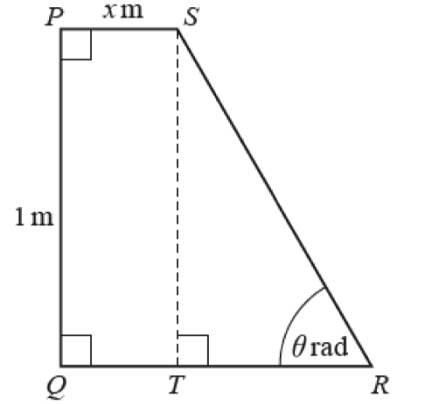# PQRS is a quadrilateral with PS parallel to QR. The perimeter of PQRS is 3 m. The length of PQ is...

## Question:PQRS is a quadrilateral with PS parallel to QR. The perimeter of PQRS is 3 m. The length of PQ is 1 m and the length of PS is x m. The point T is on QR such that ST is parallel to PQ. Angle SRT is {eq}\theta {/eq} radians.

Find an expression for x in terms of {eq}\theta {/eq}.

## Trigonometric Identities:

Trigonometric identities are used in case of right-angled triangles, they help us in relating the sides of the triangle to its angle.

For example {eq}\sin\theta = \frac{perpendicular}{hypotenuse} {/eq}

{eq}\cos\theta = \frac{base }{hypotenuse} {/eq}

and

{eq}\tan\theta = \frac{perpendicular}{base} {/eq}

{eq}\csc\theta = \frac{1}{\sin\theta} {/eq}

{eq}\sec\theta = \frac{1}{\cos\theta} {/eq}

and

{eq}\cot\theta = \frac{1}{\tan\theta} {/eq}

We can write the length of SR as {eq}\csc\theta {/eq} and the length of TR as {eq}\cot\theta {/eq}

We can see that

PQ = 1m

QT = xm as given in the figure

To find TR:

{eq}\tan \theta = \frac{1m}{TR} {/eq}

So {eq}TR = \frac{1}{\tan \theta}= \cot \theta {/eq}

{eq}\text{To find RS:} \\ \sin \theta = \frac{1m}{RS} {/eq}

RS = {eq}\frac{1}{\sin \theta} = \csc \theta {/eq}

SP = xm

So the total length of the figure by adding PQ + QT + TR + RS + SP = 1 + x + {eq}\cot\theta {/eq} + {eq}\csc\theta {/eq} + x = 3 , respectively

so the final equation becomes

{eq}2x + \csc\theta + \cot\theta = 2 {/eq}

{eq}x = \frac{2 - \csc \theta - \cot \theta}{2} {/eq}